Follow

# Management > Fleet > Cost per hour view

Updated

In order to see the Cost per hour data of your fleet, go to Management > Fleet. The following screen will be displayed: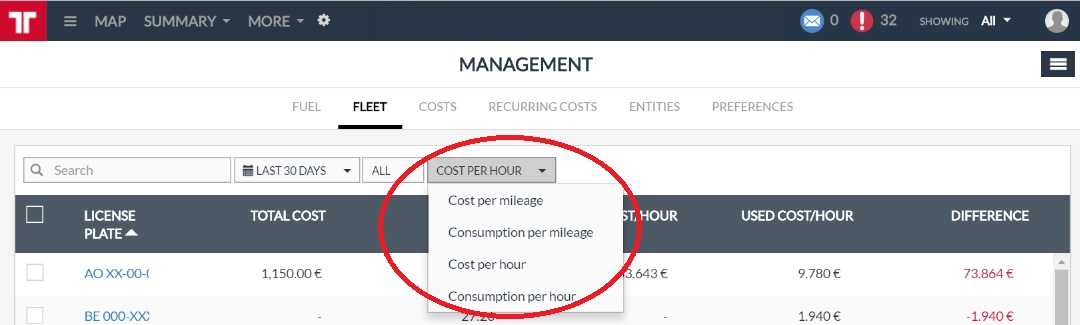On the upper part of the panel, on the View filter, select Cost per hour option. The following screen will be displayed: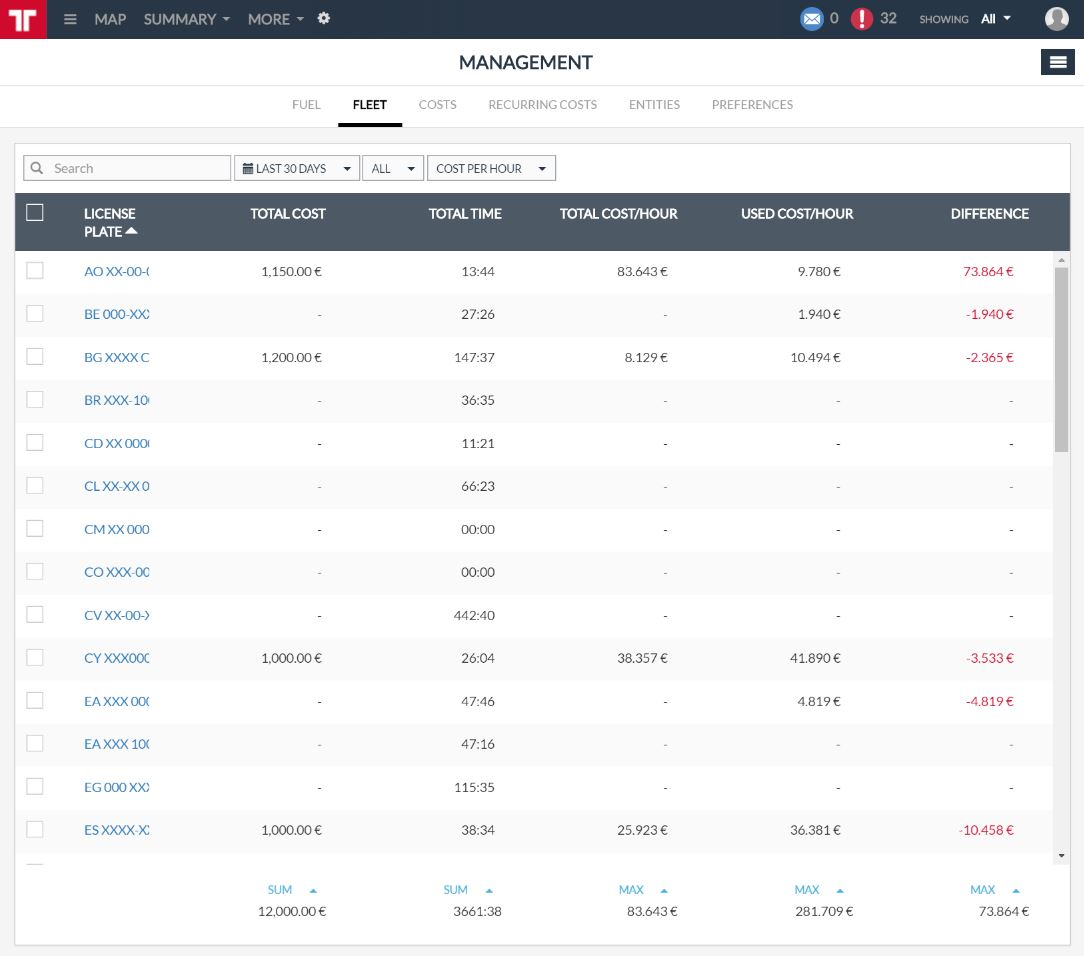It contains the following information:

 Column Description License plate Identification of the vehicle. Total cost Total cost of the vehicle in the defined period. Total time Total time with ignition ON in the defined period. Total cost/hour Consumption [L/km] = Total cost / Total time. This is the calculated cost/hour. Used cost/hour Reference value for cost/hour. Difference Difference between the calculated cost/hour and the used cost/hour in the defined period. NOTE: if the difference is higher than (+/-) 5% of the used cost/hour (used ratio), the value presented in the difference column will be in red. Otherwise, it will be in black.

At the very end of the panel, you will also have a couple of features that provide you interesting information about your fleet. For the total cost and total time columns, you will have the following mathematical operations:

• sum
• average
• count
• min
• max

For the total cost/hour, used cost/hour and difference, you will have the following mathematical operations:

• count
• min
• max

You can access them by clicking the drop-down menu at the very end of each column and selecting one of the available operations: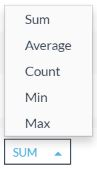After, you will see the result of the selected option, bellow the drop-down menu button: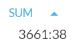The calculated ratios depend on the time periods for which they are calculated. For instance, a vehicle may have a calculated cost/hour of 50€ in August and 150€  in September. In this case, the cost/hour ratio depends on the exact cost which occurred in a period, as well as on the number of hours which the ignition was ON.

However, some organizations, like construction companies, need to have a reference value for the cost/hour ratio. This is so because they want to establish a price for their service to the customer. In Frotcom, that reference value for cost/hour is called  Used cost/hour and can be found in Administration > Vehicles > Management.

Alternatively, you can also change the vehicle's cost/hour in the Management > Fleet panel. For that, select a vehicle by ticking on the respective box on the left part of each row: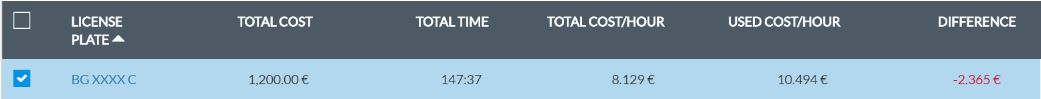After, click on the drop-down menu at the upper right part of the screen: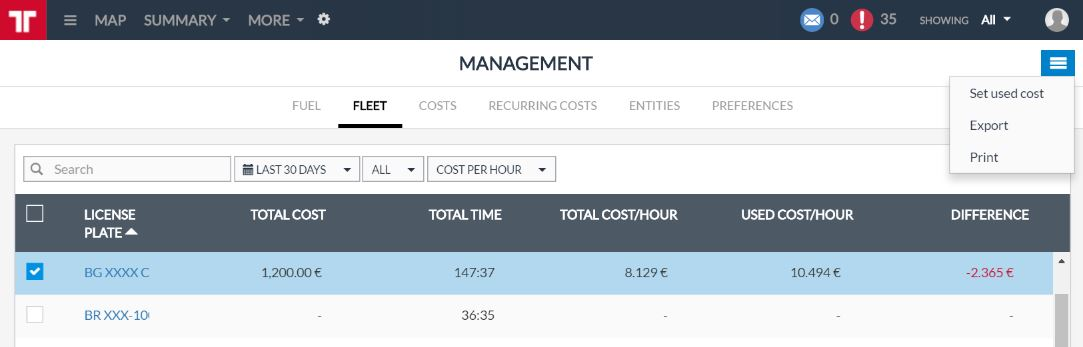The following panel will be displayed to you: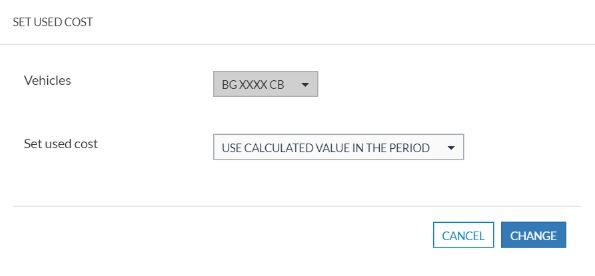On the Set used cost field, you can choose between two options for used cost consideration purposes: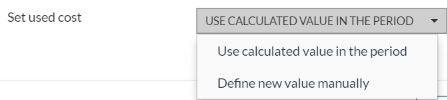Set used cost option Description Use calculated value in the period Frotcom will copy the calculated cost/hour in the used cost/hour ratio. This option is recommended when you want to accept the calculated cost/km to become your new Used cost/hour. Define new value manually After selection this option, the following field becomes available: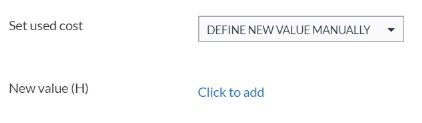Click on the Click to add link and a free text box will be available for you to define the new cost/hour: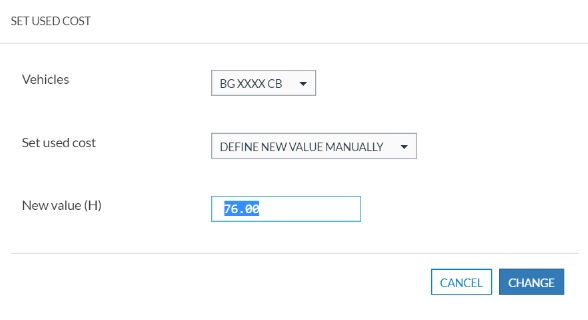When you are done with the selection of the cost/hour, click Change.

This operation can also be done for several vehicles in just one step. For that, select the vehicles before you open the Set used cost panel. In this way, you are able to set the value for more than one vehicle at a time.